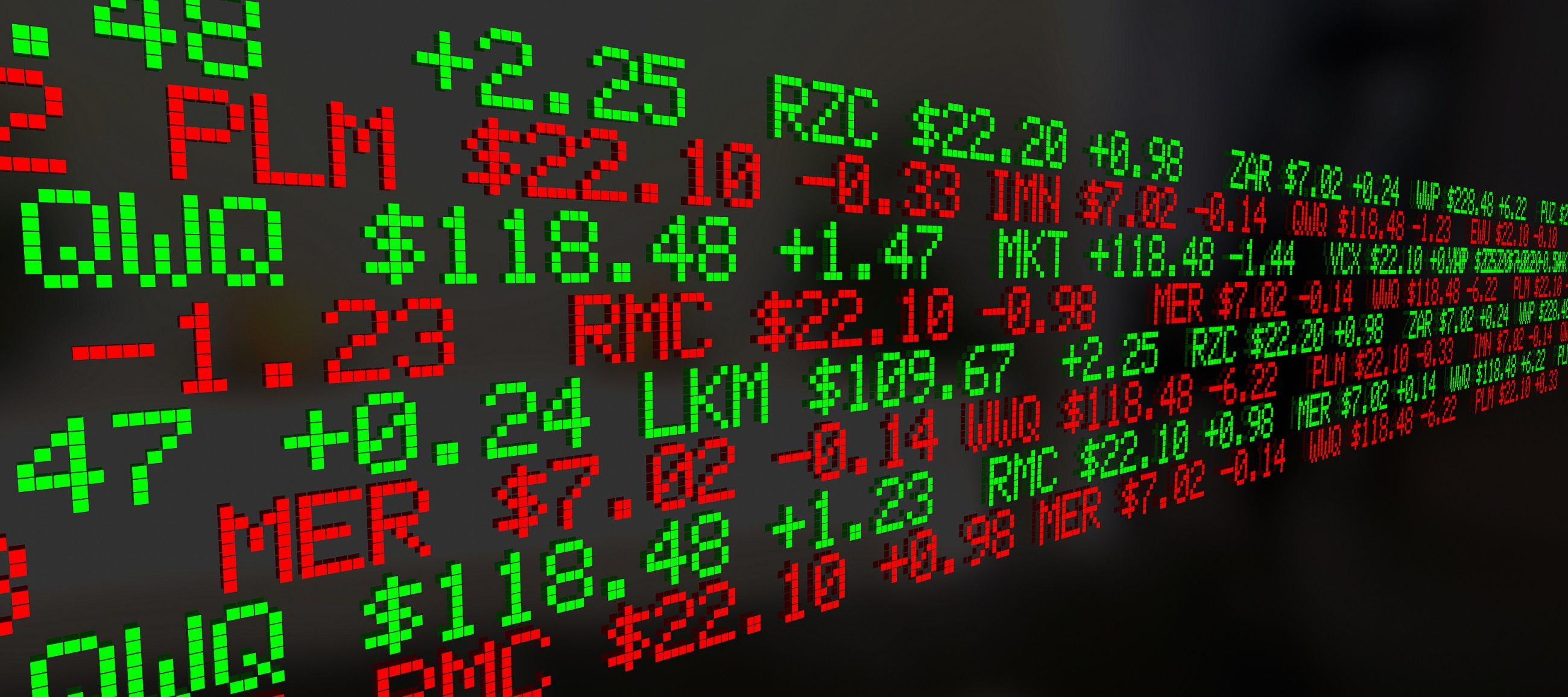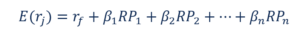# Arbitrage Pricing Theory

Forecasting the linear relationship between an asset’s returns and macroeconomic factors

## What is the Arbitrage Pricing Theory?

The Arbitrage Pricing Theory (APT) is a theory of asset pricing that holds that an asset’s returns can be forecasted with the linear relationship of an asset’s expected returns and the macroeconomic factors that affect the asset’s risk. This theory was created in 1976 by the economist, Stephen Ross. Arbitrage pricing theory offers analysts and investors a multi-factor pricing model for securities based on the relationship between a financial asset’s expected return and its risks.

The theory aims to pinpoint the fair market price of a security that may be temporarily incorrectly priced. The theory assumes that market action is less than always perfectly efficient, and therefore occasionally results in assets being mispriced – either overvalued or undervalued – for a brief period of time. However, market action should eventually correct the situation, moving price back to its fair market value. To an arbitrageur, temporarily mispriced securities represent a short-term opportunity to profit virtually risk-free.

The APT is a more flexible and complex alternative to the Capital Asset Pricing Model (CAPM). The theory provides investors and analysts with the opportunity to customize their research. However, it is more difficult to apply, as it takes a considerable amount of time to determine all the various risk factors that may influence the price of an asset.### Assumptions in the Arbitrage Pricing Theory

The Arbitrage Pricing Theory operates with a pricing model that factors in many sources of risk and uncertainty. Unlike the Capital Asset Pricing Model (CAPM) which only takes into account the single factor of the risk level of the overall market, the APT model looks at several macroeconomic factors that, according to the theory, determine the risk and return of the specific asset.

These factors provide risk premiums for investors to consider because the factors carry systematic risk that cannot be eliminated by diversifying.

The APT suggests that investors will diversify their portfolios, but that they will also choose their own individual profile of risk and returns based on the premiums and sensitivity of the macroeconomic risk factors. Risk-taking investors will exploit the differences in expected and real return on the asset by using arbitrage.

### Arbitrage in the APT

The APT suggests that the returns on assets follow a linear pattern. An investor can leverage deviations in returns from the linear pattern using the arbitrage strategy. Arbitrage is a practice of the simultaneous purchase and sale of an asset, taking advantage of slight pricing discrepancies to lock in a risk-free profit for the trade.

However, the APT’s concept of arbitrage is different from the classic meaning of the term. In the APT, arbitrage is not a risk-free operation – but it does offer a high probability of success. What the arbitrage pricing theory offers traders is a model for determining the theoretical fair market value of an asset. Having determined that value, traders then look for slight deviations from the fair market price, and trade accordingly. For example, if the fair market value of stock A is determined, using the APT pricing model, to be \$13, but the market price briefly drops to \$11, then a trader would buy the stock, based on the belief that further market price action will quickly “correct” the market price back to the \$13 a share level.

### Mathematical Model of the APT

The Arbitrage Pricing Theory can be expressed as a mathematical model:In this formula:

E(rj) – Expected return on the asset j

rf – Risk-free rate of return

ßnThe level of volatility of an asset’s price with respect to factor n, which is a macroeconomic variable causing systemic risk; how sensitive the asset is to factor n

RPn – Risk premium of factor n

Historical returns on securities are analyzed with linear regression analysis against the macroeconomic factor to estimate beta coefficients for this arbitrage pricing theory formula.

### Factors in the APT

The APT provides analysts and investors with a high degree of flexibility regarding the factors that can be applied to the model. The number and different types of factors that are used are up to an analyst’s choice. Therefore, two different investors using the APT to analyze the same security may have widely varying results when it comes to their actual trading. Even among the most devoted advocates of the theory, there is no consensus agreement of finance professionals and academics on which factors are best for predicting returns on securities.

However, Ross suggests that there are some specific factors that have shown to reliably predict price. These include sudden shifts in inflation, gross national product, and the yield curve.

Although a bit complex to work with, and something that requires time and practice to become adept at using, the Arbitrage Pricing Theory is an analytical tool that investors can use to evaluate their portfolio holdings from a basic value investing perspective, looking to identify securities that may be temporarily mispriced, well below or above their fair market value.

To learn more about evaluating securities in regard to risk vs. return, you may wish to take a look at the following related resources from CFI.

• Consumer Surplus Formula
• Law of Supply
• Return on Equity